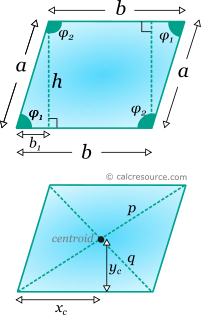Properties of a Parallelogram

This tool calculates the basic geometric properties of a parallelogram. Enter below the shape dimensions. The calculated results will have the same units as your input. Please use consistent units for any input.

 b = h = Additional input (select which): Angle φ1 Length b1 Side α α=b (rhombus) Geometric properties: Area = Perimeter = Lengths: Side α = Diagonal p = Diagonal q = Angles : deg rad φ1 = φ2 = Centroid: xc = yc =Definitions

Geometry

Parallelogram is a quadrilateral shape with two pairs of parallel sides. The area of a parallelogram is given by the formulas:

or

where a, b the lengths of the sides and h the height, perpendicular to b.

The perimeter of a parallelogram is simply the sum of the lengths of all sides:

The length of the left and right sides α, can be expressed in terms of the angle φ1, using the right triangle, with hypotenuse α (see figure below):

Interior angle φ2 is supplementary with φ1. Therefore:

There are many ways to find the lengths of diagonals, once the sides or the interior angles are known. Here, a solution employing the Pythagorean Theorem on the highlighted right triangles (see next figure) is presented:

Similarly, the other diagonal is found as:

Centroid

The centroid of parallelogram coincides with the point where diagonals cross each other. Measuring from the left vertex of base, xc and yc distances are:

where A is the area of the trapezoid.